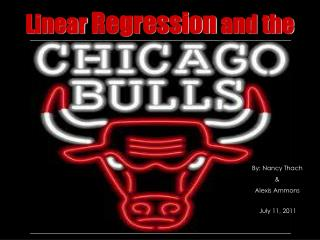Download PresentationLinear Regression and the

# Linear Regression and the - PowerPoint PPT PresentationDownload Presentation## Linear Regression and the

- - - - - - - - - - - - - - - - - - - - - - - - - - - E N D - - - - - - - - - - - - - - - - - - - - - - - - - - -
##### Presentation Transcript

1. Linear Regression and the By: Nancy Thach & Alexis Ammons July 11, 2011

2. HYPOTHESES • There is a correlation between the number of games a player played & total points scored. • There is a correlation between the age of the player & total points scored. • There is a correlation between the age of the player & salary. • There is a correlation between field goal percentage of a player & 3-point percentage. • There is a correlation between field goal attempts made by a player & field goal percentage.

3. Definitions • A Field Goal occurs when the ball enters the basket from above during play; worth 2 points, or 3 points depending on if the shooterwas standing behind the 3-point line. • A3-Point Shot is a fieldgoalworth 3 points because the shooter had both feet on the floorbehind the3-point line when he released the ball; also counts if one foot is behind the line while the other foot is in the air.

4. Source of Data: • ESPN Website: http://espn.go.com/nba/team/stats • Chicago Bulls Website: http://espn.go.com/nba/team/roster

5. r = .457 • Weak Positive Linear Correlation • Prediction: If a team member played 90 games, he would score a possible 11.2 points. • y = 0.102(90) + 2.041 • y = 11.2

6. r = .426 • Weak Negative Linear Correlation • Prediction: If a team member is 24 years old, it is estimated that he will score 10.8 points. • y = -0.724(24) + 28.21 • y = 10.8

7. r = .369 • Weak Negative Linear Correlation • Prediction: If a player is 24 years old, it is estimated that he will make about \$18,865,592 per year. • y = -47267(24) + 2E+7 = 18,865,592

8. r = .008 • Extremely weak positive correlation; line is almost horizontal. • Prediction: If a player has a field goal percentage of 0.35 = 35%, then his 3 - Point Percentage is estimated to be 0.263 = 26.3%. • y = 0.031(0.35) + 0.252 = .263

9. r = .006 • Extremely weak positive correlation; line is almost horizontal. • Prediction: If a player makes 20 Field Goal attempts, then his field goal percentage would be .465 = 46.5%. • y = 0.00007(20) + 0.464 = .465

10. Conclusions • As the number of games played increases, thetotal number of points a player scores increases. The correlation is weak. • As age increases, the total number of points that he scores decreases. The correlation is weak. • As age increases, salary decreases.The correlation is weak. • As field goal percentage increases, 3-point percentage is almost constant. The correlation is extremely weak. • As field goal attempts increases, field goal percentage is almost constant. The correlation is extremely weak.